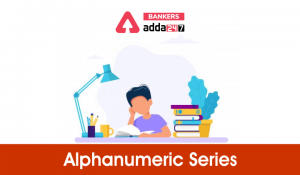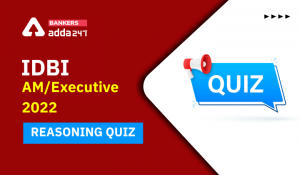Latest Banking jobs   »   Reasoning Ability Quiz For SBI Clerk...

# Reasoning Ability Quiz For SBI Clerk Prelims 2021- 11th May

Directions (1-5): Study the following information carefully to answer these questions.

M R 1 5 % ? 6 8 3 D K \$ T H 9 * F 2 P 7 # @ 4 J

Q1. How many such numbers are there in the above arrangement which are immediately followed by other number and immediately preceded by a consonant?
(a) None
(b) Three
(c) Two
(d) One
(e) None of these

Q2. How many such symbols are there in the above arrangement which are immediately followed by a symbol and also immediately preceded by a number?
(a) Two
(b) Three
(c) One
(d) Four
(e) None of these

Q3. What will come in place of ‘__’ in the following series based on the above arrangement?
M51 ?38 KHT __
(a) 72P
(b) *P2
(c) 2P*
(d) *2P
(e) None of these

Q4. Which of the following element is 15th to the left of the one which is 21st from the left end of the given arrangement?
(a) R
(b) %
(c) 5
(d) ?
(e) None of these

Q5. How many such consonant are there in the above arrangement which are immediately followed by a number but not immediately preceded by a symbol?
(a) One
(b) Two
(c) Three
(d) None
(e) None of these

Directions (6-10): Study the following sequence and answer the given questions.

K & M 3 9 U # T Q 2 ! H 7 D * 8 Y E 5 A \$ @ G S 4 5 R ^ 0 % W 1 O

Q6. Which of the following element is 10th to the left of the one which is 21st from the left end of the given arrangement?
(a) !
(b) H
(c) 2
(d) ^
(e) None of these

Q7. If all the digits are dropped from the series, which element will be tenth from the right end of the new arrangement?
(a) &
(b) @
(c) \$
(d) A
(e) None of these

Q8. How many such symbols are there in the given series which are immediately preceded by a number and immediately followed by a consonant?
(a) None
(b) One
(c) Two
(d) Three
(e) Four

Q9. How many such number are there in the given series which are immediately preceded by number and immediately followed by symbol?
(a) One
(b) Two
(c) Three
(d) More than three
(e) None of these

Q10. What should come in place of question mark (?) in the following series based on the above arrangement?
KO MW 90 ?
(a) R#
(b) K3
(c) &3
(d) #R
(e) None of these

Directions (11-15): Study the following sequence and answer the given questions.

U # N Q 2 € H 3 A @ % Z 1 P K & M 7 9 8 X E 5 B \$ * G S 4 6 R ©

Q11. If all the digits and symbols are dropped from the series, which element will be eight from the right end of the new arrangement?
(a) Z
(b) P
(c) K
(d) R
(e) None of these

Q12. How many such symbols are there in the given series which are immediately preceded by a number and immediately followed by a consonant?
(a) None
(b) One
(c) Two
(d) Three
(e) Four

Q13. What should come in place of question mark (?) in the following series based on the above arrangement?
UN2 HA% P&7 ?
(a) Z%9
(b) 1&P
(c) EB*
(d) %PZ
(e) PMK

Q14. Which among the following element will be sixth to the right of seventeenth from the right end?
(a)K
(b)&
(c)M
(d)E
(e) None of these

Q15. Which of the following elements will be third to the right of eleventh element from the left end?
(a) 5
(b) \$
(c) Z
(d) B
(e) P

Practice More Questions of Reasoning for Competitive Exams:

###### SBI Clerk Study Plan 2021

Solutions

S1. Ans.(d)
Sol. R15

S2. Ans.(a)
Sol. 7#@, 5%?

S3. Ans.(b)

S4. Ans.(d)

S5. Ans.(c)
Sol. 2P7, TH9, MR1

S6.Ans.(a)

S7.Ans.(d)

S8.Ans.(c)
Sol. Two i.e. 0%W 2!H

S9.Ans.(e)

S10.Ans.(d)

S11. Ans.(c)

S12. Ans.(b)
Sol. one i.e. 2€H

S13. Ans.(c)

S14.Ans.(d)

S15.Ans.(e)

#### Congratulations!Incorrect details? Fill the form again here

•Alphanumeric Series in Reasoning For Com...
•Reasoning Ability Quiz For IBPS RRB PO C...
•Reasoning Ability Quiz For IBPS Clerk Pr...
•Reasoning Ability Quiz For IBPS RRB PO P...
•Reasoning Ability Quiz For IDBI AM/Execu...
•Reasoning Ability Quiz For IBPS RRB PO P...